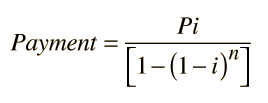# Calculation of Interest

The calculation of the interest on a loan is a useful application of algebra and exponents. For a monthly fractional interest rate i, principal P and number of payments n, the required payment may be calculated fromNote that in this formula, i is not an annual percentage rate, but a monthly fractional interest rate. For example, if the annual rate is 12%, the monthly fractional interest rate is i = 0.01 . For purposes of calculation, the active formula below may be used by entering the relevant data and then clicking on the quantity you wish to calculate.Justin Cantrell

For a loan of principal P = \$
for a term of n = months = years
at interest rate i = % annual rate,
the monthly payment is \$

For this loan the total amount repaid is \$
and the total interest paid is \$

Note: The interest rate calculation is not yet implemented. The equation for the interest is said to be "transcendental" in that it cannot be solved in closed form. An interative calculation is required.

Index

 HyperPhysics*****HyperMath*****Algebra R Nave
Go Back